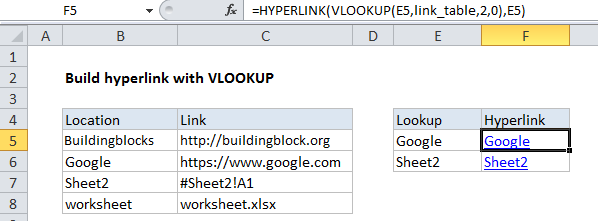# Create hyperlink with VLOOKUP in Excel

To create a hyperlink from a lookup, you can use the VLOOKUP function together with the HYPERLINK function.

The hyperlink function allows you to create a working link with a formula. It takes two arguments: link_location and, optionally, friendly_name.

Worked Example:   Vlookup Examples in Excel

## Formula

`=HYPERLINK(VLOOKUP(name,table,column,0),name)`## Explanation

In the example shown, the formula in F5 is:

`=HYPERLINK(VLOOKUP(E5,link_table,2,0),E5)`

### How this formula works

Working from the inside out, VLOOKUP looks up and retrieves a link value from column 2 of the named range “link_table” (B5:C8). The lookup value comes from column E, and VLOOKUP is configured for exact match.

Worked Example:   How to use Excel VLOOKUP Function

The result is fed into HYPERLINK as link_location, and the text in column E is used for friendly_name.

Worked Example:   Dynamic lookup table with INDIRECT in Excel